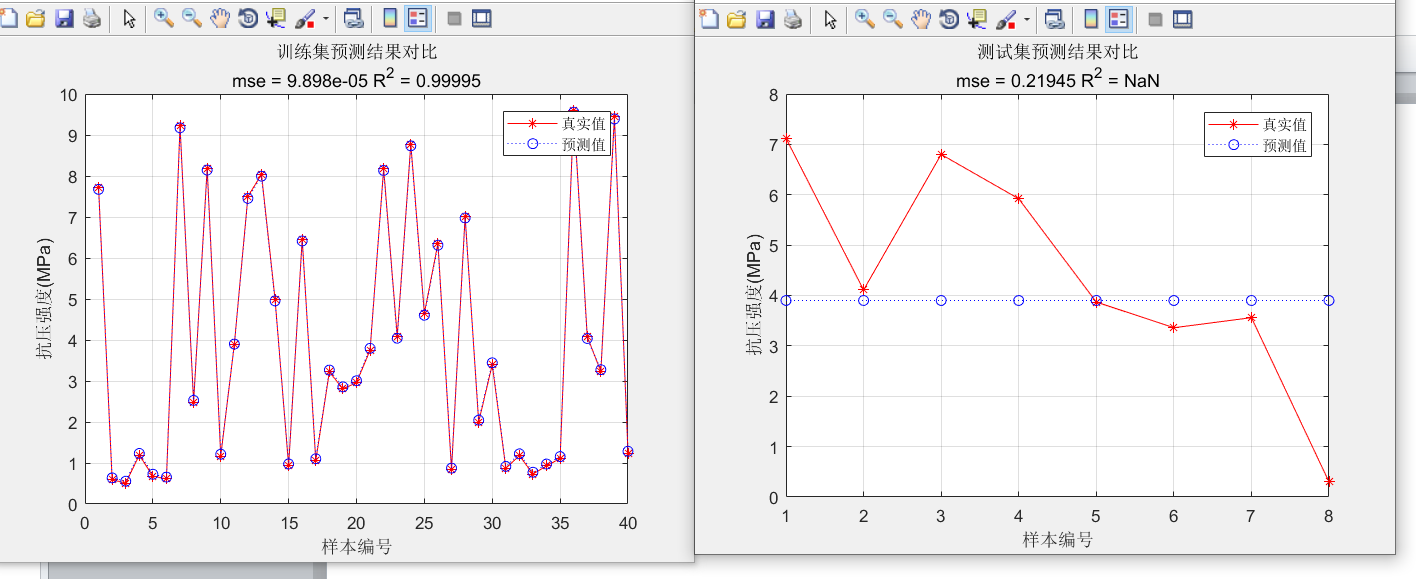Matlab中libsvm工具箱用PSO优化SVM做回归，训练集结果很好，但测试集结果是一条直线？%%
% 1. 随机产生训练集和测试集
n = randperm(size(c,2));

%%
% 2. 训练集——40个样本
p_train = c(:,n(1:40))';
t_train = d(:,n(1:40))';
%%
% 3. 测试集——8个样本
p_test = c(:,n(41:end))';
t_test = d(:,n(41:end))';

%% III. 数据归一化
%%
% 1. 训练集
[pn_train,inputps] = mapminmax(p_train');
pn_train = pn_train';
pn_test = mapminmax('apply',p_test',inputps);
pn_test = pn_test';

%%
% 2. 测试集
[tn_train,outputps] = mapminmax(t_train');
tn_train = tn_train';
tn_test = mapminmax('apply',t_test',outputps);
tn_test = tn_test';
%% 参数初始化

``````c1 = 1.5; % c1 belongs to [0,2]
c2 = 1.5; % c2 belongs to [0,2]
maxgen=200;   % 进化次数
sizepop=50;   % 种群规模
popcmax=10^(3);
popcmin=10^(-5);
popgmax=10^(3);
popgmin=10^(-5);
k = 0.02; % k belongs to [0.1,1.0];
Vcmax = k*popcmax;
Vcmin = -Vcmax ;
Vgmax = k*popgmax;
Vgmin = -Vgmax ;
``````

% SVM参数初始化
v = 5;
%% 产生初始粒子和速度

``````for i=1:sizepop
pop(i,1) = (popcmax-popcmin)*rand+popcmin;    % 初始种群
pop(i,2) = (popgmax-popgmin)*rand+popgmin;
V(i,1)=Vcmax*rands(1);  % 初始化速度
V(i,2)=Vgmax*rands(1);
cmd = ['-v ',num2str(v),' -t 2',' -c ',num2str( pop(i,1) ),' -g ',num2str(pop(i,2) ),' -s 3 -p 0.1'];
fitness(i) = svmtrain(tn_train, pn_train, cmd);
fitness(i) = -fitness(i);
end
[global_fitness bestindex]=min(fitness); % 全局极值
local_fitness=fitness;   % 个体极值初始化
global_x=pop(bestindex,:);   % 全局极值点
local_x=pop;    % 个体极值点初始化
``````

tic
%% 迭代寻优

``````for i=1:maxgen

for j=1:sizepop

%速度更新
wV = 0.4; %
V(j,:) = wV*V(j,:) + c1*rand*(local_x(j,:) - pop(j,:)) + c2*rand*(global_x - pop(j,:));
if V(j,1) > Vcmax
V(j,1) = Vcmax;
end
if V(j,1) < Vcmin
V(j,1) = Vcmin;
end
if V(j,2) > Vgmax
V(j,2) = Vgmax;
end
if V(j,2) < Vgmin
V(j,2) = Vgmin;
end

%种群更新
wP = 0.6;
pop(j,:)=pop(j,:)+wP*V(j,:);
if pop(j,1) > popcmax
pop(j,1) = popcmax;
end
if pop(j,1) < popcmin
pop(j,1) = popcmin;
end
if pop(j,2) > popgmax
pop(j,2) = popgmax;
end
if pop(j,2) < popgmin
pop(j,2) = popgmin;
end

``````4 天之前 回复